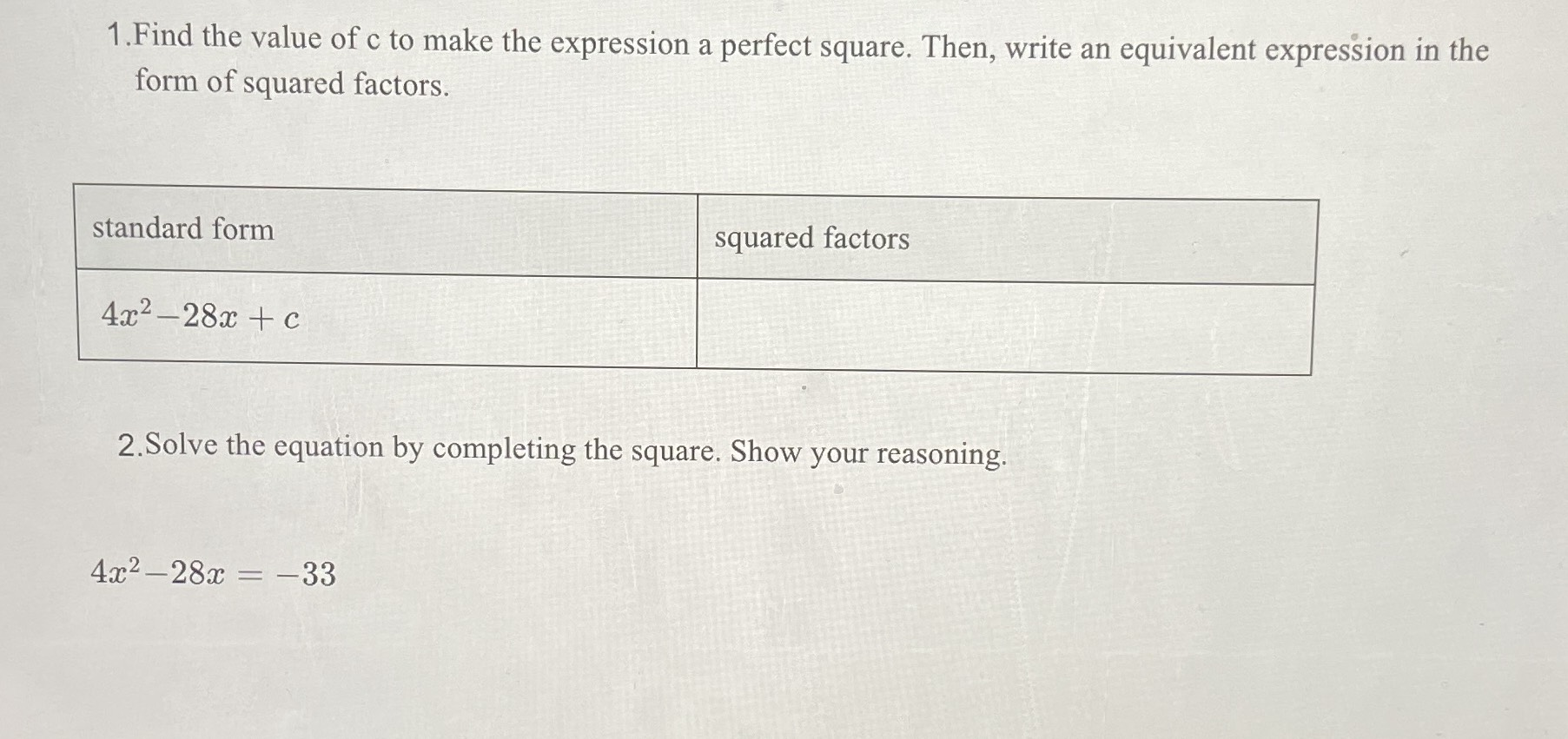### ¿Todavía tienes preguntas de matemáticas?

Pregunte a nuestros tutores expertos
Algebra
Pregunta1. Find the value of c to make the expression a perfect square. Then, write an equivalent expression in the form of squared factors.

standard form

$$4 x ^ { 2 } - 28 x + c$$

2. Solve the equation by completing the square. Show your reasoning.

$$4 x ^ { 2 } - 28 x = - 33$$

1) c = 49 , $$(2x- 7)^2$$ ,
2) $$(2x- 7)^2= 4^2, x = 5.5,1.5$$